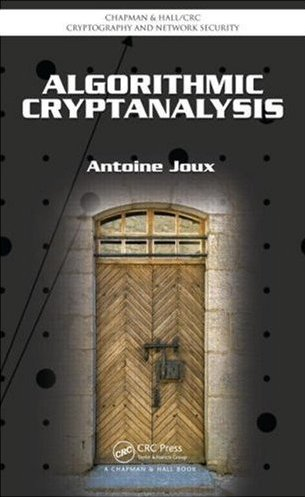### Algorithmic Cryptanalysis

• Libraries & Programs
• IACR
• Cryptographer's world
• CRC Press
• Book on CRC Press

# Hints for Exercises (Chapter 14)

• Exercise 1: Try brute force for the order of the curve. For singular curves, identify the cases where the addition formulas cannot be used.
• Exercise 4: Look at the degree of the polynomials.
• Exercise 6: Use the inverse map and bilinearity to move multipliers from right to left. Show how to take powers of multipliers.
• Exercise 8: For a given curve modulo p, there are many possible curves modulo p-squared.

Credits for page styles: Dynamic Drive CSS Library